# Rust – For and Range

• Last Updated : 28 Feb, 2022

Suppose you have a list of items and you want to iterate over each item in the list. One thing we can do is use a for loop. Using a for loop, we can iterate over a list of items. In Rust, we use the keyword for in followed by the variable name or the range of items we want to iterate over.

Let’s see an example of a for loop.

Suppose we have a list of numbers and we want to iterate over each number in the list.

## Rust

 `let` `numbers = [1, 2, 3, 5]; ` `for` `i ``in` `numbers { ` `   ``println!(``"{}"``, i); ` `}`

Here, for is the keyword that we use to iterate over a list of items. The variable name is i. The list of items is numbers. This will print out each number in the list.

Output:

```1
2
3
5```

### For and Range:

A for loop has the following structure:

```for iterator in range or vector{
//    statement
}```

Here, we use for loop to do the repetitive task. A for loop always starts with the keyword for, we can use for loop instead of while loop to make our code look cleaner. The iterator can be any variable. The range in Rust is similar to the range function in the Python language. Using range, we can define a range of numbers. For example, we can define a range of numbers from 1 to 10 using the following syntax:

`0..11`

Here, the notation a..b is used to define the range. The range is inclusive of the start and exclusive of the end. And if we want to make the range inclusive of the end, we can use the range syntax a..=b.

Example 1:

## Rust

 `// Using for and range in Rust ` `fn` `main() { ``// main function ` ` `  `// define a range of numbers from 20 to 30 ` `   ``for` `i ``in` `20..31 { ``// for loop ` `       ``println!(``"The number is {}"``, i); ``// print the number ` `   ``} ``// end of for loop ` `} ``// end of main function`

Output: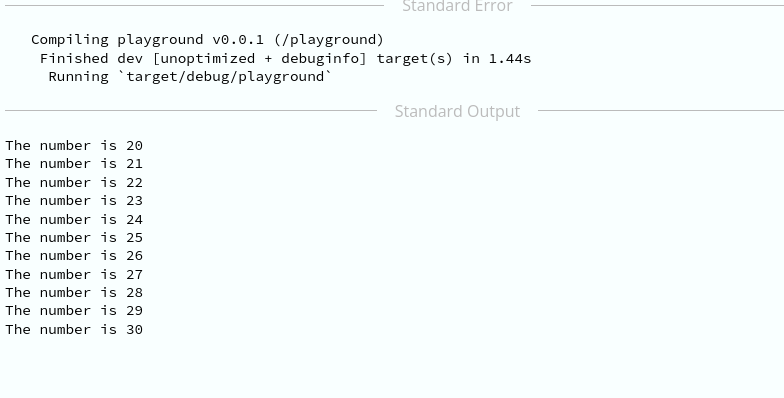for loop with range

Example 2:

## Rust

 `// using range and for loop ` `fn` `main() { ``// main function ` `   `  `  ``let` `colors = [``"red"``, ``"green"``, ``"blue"``]; ``// define a list of colors ` ` `  `  ``// iterate over the list of colors ` `    ``for` `i ``in` `0..colors.len() { ``// for loop ` `           ``println!(``"The color name is {}"``, colors[i]); ``// print the color name ` ` `  `   ``} ``// end of for loop ` `} ``// end of main function`

Output: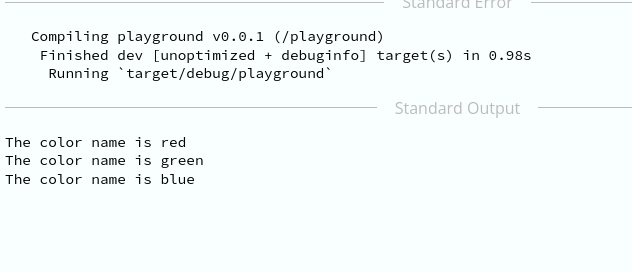range and len()

Here, we 0..colors.len() is the range of colors. The range is inclusive of the start and exclusive of the end. Here, the number of colors is 3. lThe len() is a method of the String type and it returns the number of strings in the list.

Example 3:

## Rust

 `// Using for loop and vectors in Rust ` `fn` `main() { ``// main function ` `   ``let` `countries = vec![``"India"``, ``"China"``, ``"USA"``, ``"Russia"``]; ``// define a vector of countries ` ` `  `   ``for` `country ``in` `countries.iter() { ``// for loop ` `       ``println!(``"The country name is {}"``, country); ``// print the country name ` `   ``} ``// end of for loop ` `} ``// end of main function`

Output: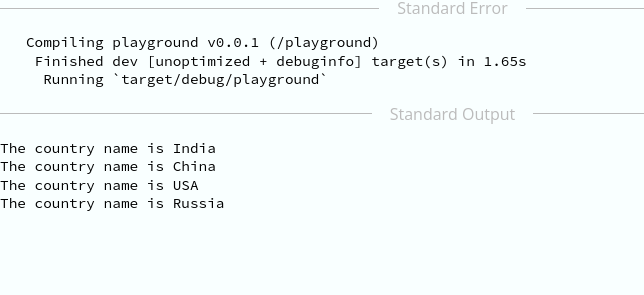range and iter()

Here, the iter() is a method of the vector type and it returns an iterator. The country is a variable name and it is used to iterate over the vector. In general, the iter() borrows each element of the vector through each iteration.

Example 4:

## Rust

 `//  using enumerate and for loop in Rust ` `fn` `main() { ``// main function ` `   ``let` `programming_languages = [``"Rust"``, ``"Python"``, ``"Java"``, ``"C++"``]; ``// define a vector of programming languages ` ` `  `   ``for` `(index, language) ``in` `programming_languages.iter().enumerate() { ``// for loop ` ` `  `       ``println!(``"The programming language at index {} is {}"``, index, language); ``// print the programming language at index ` ` `  `   ``} ``// end of for loop ` `} ``// end of main function`

Output: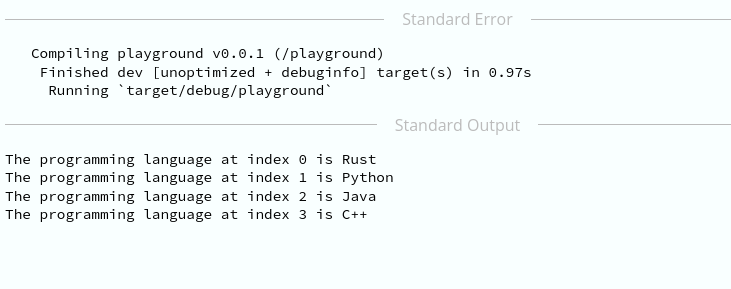using enumerate()

Here, we have used enumerate() method of the vector type. The enumerate() method allows us to iterate over the vector and also to get the index of the element. The index is 0-based, which means the starting index in enumerating is 0, not 1.

Example 5:

## Rust

 `// Using for loop with step_by() in Rust ` `fn` `main() { ``// main function ` `   ``let` `programming_languages = [``"Rust"``, ``"Python"``, ``"Java"``, ``"C++"``]; ``// define a vector of programming languages ` ` `  `   ``for` `(index, language) ``in` `programming_languages.iter().enumerate().step_by(2) { ``// for loop ` `       ``println!(``"The programming language at index {} is {}"``, index, language); ``// print the programming language at index ` ` `  `   ``} ``// end of for loop ` `} ``// end of main function`

Output: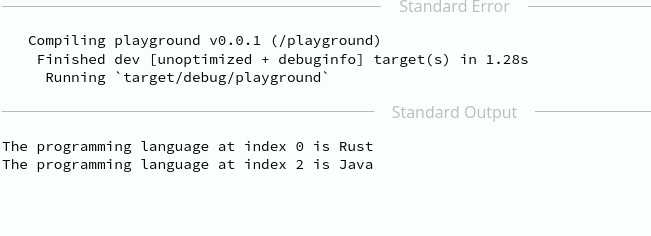Using step_by()

Here, the step_by() method allows us to iterate over the vector with a step size. In this case, the step size is 2.

My Personal Notes arrow_drop_up
Recommended Articles
Page :